# set_dataframe() : Pandas DataFrame to Google sheets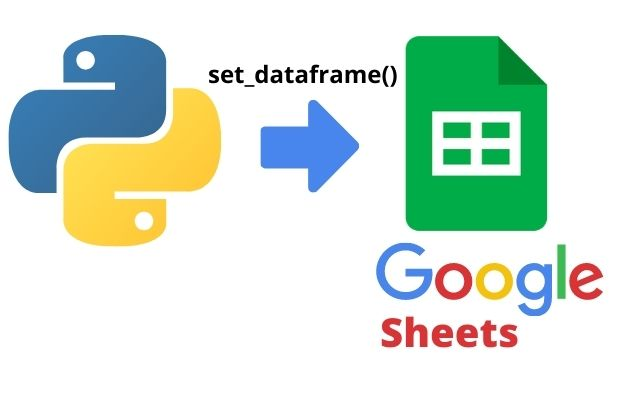Inserting Pandas DataFrame to google sheets by using set_dataframe() with options to copy index

Create one folder inside your google drive, give the name as python-test.
Give Share authorization ( Share with people and group ) to Google API service account you are using.

Creating one new File my_sheet1 ( google sheet ) inside python-test directory.
``````import pygsheets
gc=pygsheets.authorize(service_account_file=path)
sh=gc.create('my_sheet1',folder_name='python-test')``````

## set_dataframe

``````set_dataframe(df, start, copy_index=False, copy_head=True,
extend=False, fit=False, escape_formulae=False, **kwargs)``````
OptionsDetails
dfPandas DataFrame
startStaring Point tuple ( row, column). It is (1,1) in below example
copy_indexDefault False, Copy Index data from DataFrame
copy_headTrue ( default ), column headers of Pandas DataFrame is copied ( see example below ).
extendFalse( default) If required add columns and rows ( see example )
fitFalse(default), Resize to fit all data in DataFrame if required ( see example)
escape_formulaeHow to handle formula ( value with = or +- sign ) ( see example below )
nanReplace NaN with input value ( see example below )
Create Pandas DataFrame and then store the same inside our google sheets my_sheets1. Here we have used one blank google sheet and given permission to service account before running this code.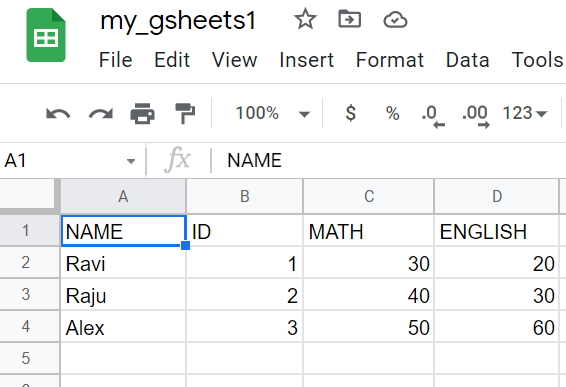``````import pygsheets
gc=pygsheets.authorize(service_account_file=path)
sh=gc.open('my_gsheets1') # open the existing file
wk1=sh # first worksheet
#wk1.clear() # to remove all data from sheet
import pandas as pd # using pandas library
my_dict={
'NAME':['Ravi','Raju','Alex'],
'ID':[1,2,3],'MATH':[30,40,50],
'ENGLISH':[20,30,60]
} # Create dictionary
df = pd.DataFrame(data=my_dict) # Create DataFrame using dictionary
wk1.set_dataframe(df,(1,1)) # Place DataFrame from row 1 column 1
print(wk1.get_row(4,include_tailing_empty=False)) # for testing ``````
Output ( for last line )
``['Alex', '3', '50', '60']``

## copy_index

Let us add the DataFrame with index.
``wk1.set_dataframe(df,(1,1),copy_index=True) #``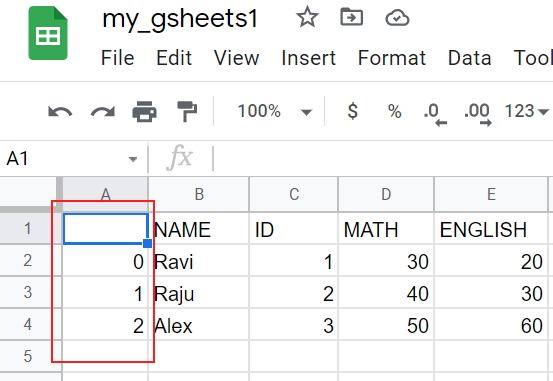``wk1.set_dataframe(df,(1,1),copy_index=True, copy_head=False) ``## extend

If DataFrame has more rows and columns than the sheet , then it will generate error saying exceeds grid limits. Max rows: 3, max columns: 5.

However we can use extend option with True ( default is False ) to expand the sheet to match the DataFrame rows and columns. We are reducing the default rows and columns of the sheet by using resize()
``````wk1.resize(2,2)
wk1.set_dataframe(df,(1,1),copy_index=True, extend=True)``````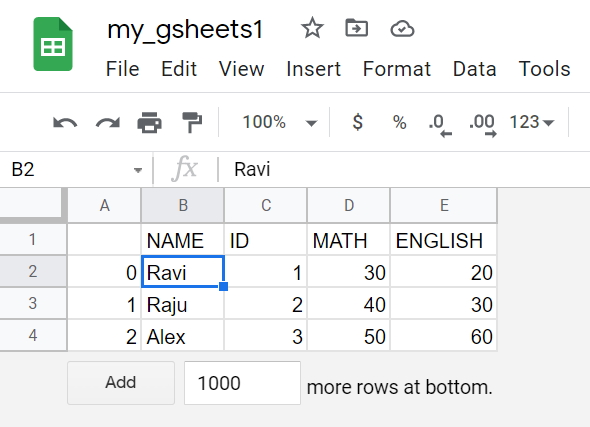## fit

Our sheet has 10 rows and 10 columns and our DataFrame has 5 rows and 4 columns. We can shrink our sheet to exactly match the size of our DataFrame and don't want to leave any blank cell outside the DataFrame by using fit option.
``````wk1.resize(10,10) # resize rows and columns of sheet
wk1.set_dataframe(df,(1,1),copy_index=True, fit=True) ``````## escape_formulae

We will change the input DataFrame to include a formula like this `'=40+5'`. Default value for escape_Formulae is False.
``````my_dict={
'NAME':['Ravi','Raju','Alex'],
'ID':[1,2,3],'MATH':[30, '=40+5',50],
'ENGLISH':[20,30,60]
} # Create dictionary
df = pd.DataFrame(data=my_dict) # Create DataFrame using dictionary
wk1.set_dataframe(df,(1,1), escape_formulae=False)``````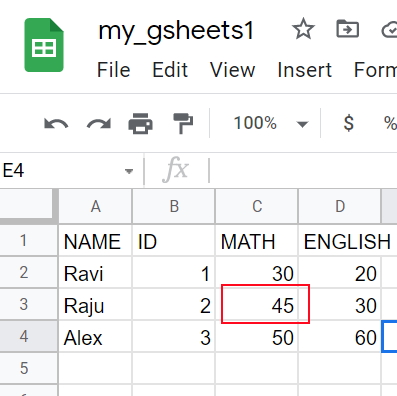Let us change the escape_formulae option to True
``wk1.set_dataframe(df,(1,1), escape_formulae=True)``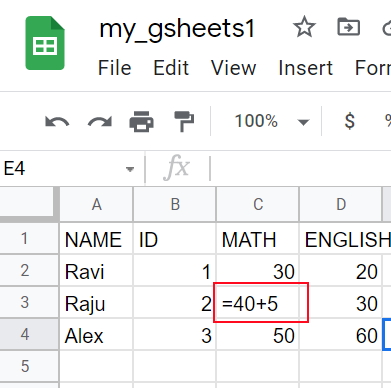## nan

We can replace the NaN with values. Here we are using Numpy to add some NaN values to our DataFrame. Check the np.NaN data
``````import pandas as pd # using pandas library
import numpy as np
my_dict={'NAME':['Ravi','Raju','Alex',None,'King',None],
'ID':[1,2,np.NaN,4,5,6],
'MATH':[80,40,70,70,82,30],
'ENGLISH':[81,70,40,50,np.NaN,30]}
df = pd.DataFrame(data=my_dict) # Create DataFrame using dictionary
wk1.set_dataframe(df,(1,1))``````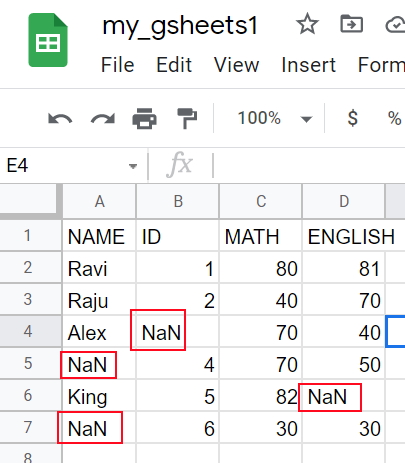We will add nan option with * value.
``wk1.set_dataframe(df,(1,1), nan='*') ``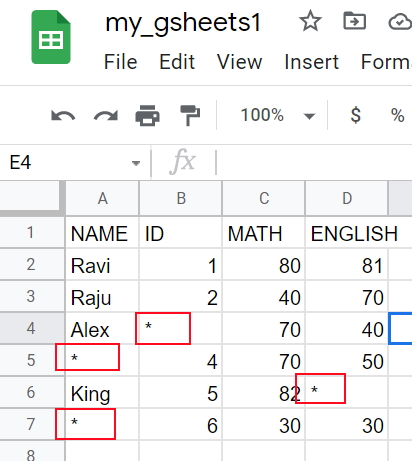Subscribe to our YouTube Channel here

## Subscribe

* indicates required
Subscribe to plus2netplus2net.com